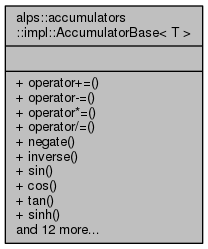ALPSCore reference
alps::accumulators::impl::AccumulatorBase< T > Class Template Reference

#include <feature.hpp>

Collaboration diagram for alps::accumulators::impl::AccumulatorBase< T >:## Public Types

typedef T value_type

typedef ResultBase< T > result_type

## Public Member Functions

template<typename U >
void operator+= (U)

template<typename U >
void operator-= (U)

template<typename U >
void operator*= (U)

template<typename U >
void operator/= (U)

void negate ()

void inverse ()

void sin ()

void cos ()

void tan ()

void sinh ()

void cosh ()

void tanh ()

void asin ()

void acos ()

void atan ()

void abs ()

void sq ()

void sqrt ()

void cb ()

void cbrt ()

void exp ()

void log ()

## Detailed Description

### template<typename T> class alps::accumulators::impl::AccumulatorBase< T >

Definition at line 128 of file feature.hpp.

## Member Typedef Documentation

template<typename T >
 typedef ResultBase alps::accumulators::impl::AccumulatorBase< T >::result_type

Definition at line 131 of file feature.hpp.

template<typename T >
 typedef T alps::accumulators::impl::AccumulatorBase< T >::value_type

Definition at line 130 of file feature.hpp.

## Member Function Documentation

template<typename T >
 void alps::accumulators::impl::AccumulatorBase< T >::abs ( )
inline

Definition at line 161 of file feature.hpp.

template<typename T >
 void alps::accumulators::impl::AccumulatorBase< T >::acos ( )
inline

Definition at line 159 of file feature.hpp.

template<typename T >
 void alps::accumulators::impl::AccumulatorBase< T >::asin ( )
inline

Definition at line 158 of file feature.hpp.

template<typename T >
 void alps::accumulators::impl::AccumulatorBase< T >::atan ( )
inline

Definition at line 160 of file feature.hpp.

template<typename T >
 void alps::accumulators::impl::AccumulatorBase< T >::cb ( )
inline

Definition at line 164 of file feature.hpp.

template<typename T >
 void alps::accumulators::impl::AccumulatorBase< T >::cbrt ( )
inline

Definition at line 165 of file feature.hpp.

template<typename T >
 void alps::accumulators::impl::AccumulatorBase< T >::cos ( )
inline

Definition at line 153 of file feature.hpp.

template<typename T >
 void alps::accumulators::impl::AccumulatorBase< T >::cosh ( )
inline

Definition at line 156 of file feature.hpp.

template<typename T >
 void alps::accumulators::impl::AccumulatorBase< T >::exp ( )
inline

Definition at line 166 of file feature.hpp.

template<typename T >
 void alps::accumulators::impl::AccumulatorBase< T >::inverse ( )
inline

Definition at line 148 of file feature.hpp.

template<typename T >
 void alps::accumulators::impl::AccumulatorBase< T >::log ( )
inline

Definition at line 167 of file feature.hpp.

template<typename T >
 void alps::accumulators::impl::AccumulatorBase< T >::negate ( )
inline

Definition at line 145 of file feature.hpp.

template<typename T >
template<typename U >
 void alps::accumulators::impl::AccumulatorBase< T >::operator*= ( U )
inline

Definition at line 139 of file feature.hpp.

template<typename T >
template<typename U >
 void alps::accumulators::impl::AccumulatorBase< T >::operator+= ( U )
inline

Definition at line 133 of file feature.hpp.

template<typename T >
template<typename U >
 void alps::accumulators::impl::AccumulatorBase< T >::operator-= ( U )
inline

Definition at line 136 of file feature.hpp.

template<typename T >
template<typename U >
 void alps::accumulators::impl::AccumulatorBase< T >::operator/= ( U )
inline

Definition at line 142 of file feature.hpp.

template<typename T >
 void alps::accumulators::impl::AccumulatorBase< T >::sin ( )
inline

Definition at line 152 of file feature.hpp.

template<typename T >
 void alps::accumulators::impl::AccumulatorBase< T >::sinh ( )
inline

Definition at line 155 of file feature.hpp.

template<typename T >
 void alps::accumulators::impl::AccumulatorBase< T >::sq ( )
inline

Definition at line 162 of file feature.hpp.

template<typename T >
 void alps::accumulators::impl::AccumulatorBase< T >::sqrt ( )
inline

Definition at line 163 of file feature.hpp.

template<typename T >
 void alps::accumulators::impl::AccumulatorBase< T >::tan ( )
inline

Definition at line 154 of file feature.hpp.

template<typename T >
 void alps::accumulators::impl::AccumulatorBase< T >::tanh ( )
inline

Definition at line 157 of file feature.hpp.

The documentation for this class was generated from the following file: